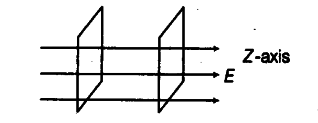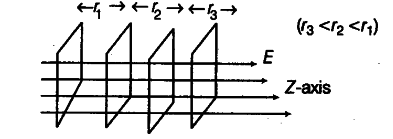# Describe schematically the equipotential surfaces corresponding to

Describe schematically the equipotential surfaces corresponding to
(i) a constant electric field in the Z-direction,
(ii) a field that uniformly increases in magnitude but remains in a constant (say Z) direction and
(iii) a single positive charge at the origin.

(i) As the constant electric field in the Z-direction, the equipotential surfaces are normal to the field, i.e. in XY-plane. The equipotential surfaces are equidistant from each other.(ii) As, the electric field increases in the direction of Z-axis, the equipotential surface is normal to Z-axis, i.e. in XY-plane and they become closer and closer as the field increases.(iii) As a single positive charge is placed at origin, the equipotential surfaces are concentric spheres with origin at centre.